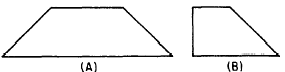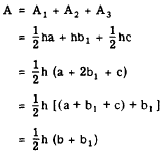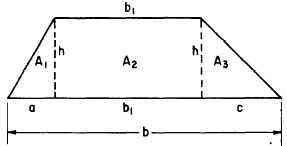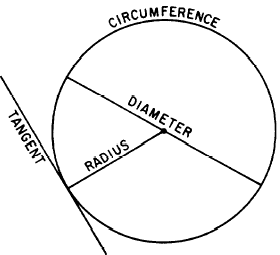TrapezoidsCustom SearchTrapezoids A TRAPEZOID is a quadrilateral in which two sides are parallel and the other two sides are not parallel. By orienting a trapezoid so that its parallel sides are horizontal, we may call the parallel sides bases. Observe that the bases (See fig. 17-15.)Figure 17-15.-Typical trapezoids. The area of a trapezoid may be found by separating it into two triangles and a rectangle, as in figure 17-16. The total area A of the trapezoid is the sum of A1 plus A2 plus A3, and is calculated as follows:Thus the area of a trapezoid is equal to onehalf the altitude times the sum of the bases.Figure 17-16.-Area of a trapezoid. Practice problems. Find the area of each of the following figures: 1. Rhombus; base 4 in., altitude 3 in. 2. Rectangle; base 6 ft, altitude 4 ft 3. Parallelogram; base 6 ft and 4 ft, altitude 2 yd. Answers: 1. 12 sq in.  2. 24 sq ft  3. 40 sq yd 4. 30 sq ft CIRCLES The mathematical definition of a circle states that it is a plane figure bounded its diameter. Parts of a Circle The CIRCUMFERENCE of a circle is the line that forms its outer boundary. Circumference is the special term used in referring to radius at the point of tangency.Figure 17-17.- Parts of a circle. An ARC is a portion of the circumference of a circle. A CHORD is a straight line joining the end points of any arc. The portion of the area of a circle cut off by a chord is a SEGMENT of the circle, and the portion of the circle’s area cut off by two radii (radius lines) is a SECTOR. (See fig. 17-18.)Integrated Publishing, Inc. - A (SDVOSB) Service Disabled Veteran Owned Small Business